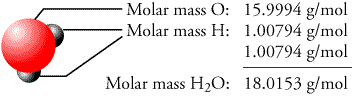Topic 14, Acids, Bases, and Salts
Topic 14, Acids, Bases, and Salts
(Key concepts)Tables K (common acids), L (common bases), M (acid-base indicators), E (lists hydronium and hydroxide ion; lists polys that may be present in salts; lists (-) polys that may be present in acids), R (organic acids, -COOH), and J (find H2, metals below will NOT react with acids to produce H2 gas, metals above WILL react with acids to produce H2 gas).

Acids:
give off H+ (or H3O+) ions
(donate H+)
pH < 7
sour
change the color of acid-base indicators
acids turn litmus red
acids turn phth colorless
electrolytes (due to mobile H+ (H30+) ions
neutralize bases
acid + base -->  water + salt
react with certain metals to produce H2(g)
HCl, H2SO4, CH3COOH, etc.Bases:
give off OH- ions
(accept H+)
pH > 7
bitter
change the color of acid-base indicators
base turn litmus blue
bases turn phth pink
electrolytes (due to mobile OH- ions)
neutralize acids
acids + base -->  water + salt
slippery
NaOH, Mg(OH)2, NH3, etc.Salts:
m + n; NaCl
m + -p, NaNO3
+p + n, NH4Cl
+p + -p, NH4NO3
electrolytes (due to dissociation)pH scale:
0 to 14
7 = neutral
acids < 7
bases > 7
each move tenfold increase or decreaseArrhenius Acids & Bases:
acids give off H+ (H3O+) as the only (+) ion in solution
bases give off OH- ion as the only (-) ion in solution

Neutralization:
acid + base -->  water + salt
double replacement reaction
follows AB  +  CD  -->  AD  +  CB
HCl  +  NaOH  -->  HOH  +  NaCl
H+  +  OH-  -->  HOH (H2O)

Molarity:
M = moles of solute / liters of solution
Table T
1M is less concentrated than 6MMolar Mass:
NaCl = 23 + 35 = 58 grams/moleTitration:
MAVA = MBVB
Table T